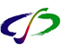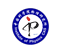引用本文: 郑伟谋. 量纲分析和量纲制[J]. 物理, 2021, 50(12): 797-811.ZHENG Wei-Mou. Dimensional analysis and dimension systems[J]. PHYSICS, 2021, 50(12): 797-811.
 Citation: ZHENG Wei-Mou. Dimensional analysis and dimension systems[J]. PHYSICS, 2021, 50(12): 797-811.## Dimensional analysis and dimension systems

• 摘要: 物理定律不依赖于测量单位的选择。量纲分析探讨这种不变性及其后果和应用。无纲量为单位变换下的不变量，物理规律最终必然只能用无纲量表达。从一个问题中的物理变量可构造出的无纲量数要少于原始变量数，带来简化，构造的无纲量可更深刻反映物理量间的内在关系。量纲概念足够深刻，但方法足够简单，应该是物理训练的重要内容。文章阐述量纲分析的基本概念、原理及其应用，大部分内容来自文献，着重讨论量纲制及其与单位制的关系，企图厘正文献中的一些混乱。特别指出，仅就量纲分析操作而言，可以只用MLT量纲制。

Abstract: Physical rules are independent of measuring units. Dimensional analysis explores such invariance, its outcomes and applications. Dimensionless quantities are the invariants under changes of units. Physical rules must ultimately be presented only in dimensionless quantities. The number of dimensionless quantities that can be extracted in a problem is less than that of the original variables, which results in simplification. The dimensionless quantities such extracted may deeply reflect the intrinsic relation among physical variables. The concept of dimension is profound, but its use is simple, so dimensional analysis should be an important section of physics training. Some fundamental concepts and principles involving dimensional analysis and its applications are expounded, including dimension systems and their relation to units systems. Examples are taken from the literature, where there is still some confusion, which I shall attempt to clarify. It is emphasized that, when applying dimensional analysis, we can just use the mass-length-time dimension system./下载:  全尺寸图片 幻灯片
• 分享
• 用微信扫码二维码

分享至好友和朋友圈